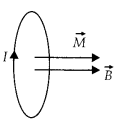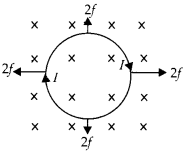Enlightened

# Question 25: NCERT Solutions for 12th Class Physics: Chapter 4-Moving Charges and Magnetism

• 0

Question 25: NCERT Solutions for 12th Class Physics: Chapter 4-Moving Charges and Magnetism

A circular coil of 20 turns and radius 10 cm is place in a uniform magnetic field of 0.10 T normal to the plane of the coil. If the current in the coil is 5.0 A, what is the
(a) total torque on the coil,
(b) total force on the coil,
(c) average force on each electron in the coil due to the magnetic field?
(The coil is made of copper wire of cross-sectional area 10-5 m2, the free electron density in copper is given to be about 1029   m-3.)

Share

1. Solution:
The magnetic field is normal to the plane of the coil, so condition of minimum torque.(a) Torque on the coil τ = NIB A sinθ here θ = 0° τ = 0
(b) Force on every element of the coil is cancelled by force on corresponding element. Net force on the unit is zero.(c) To calculate force on each electron, let us find drift velocity.Check the complete chapter with solutions.

NCERT Solutions for 12th Class Physics: Chapter 4-Moving Charges and Magnetism

• 0